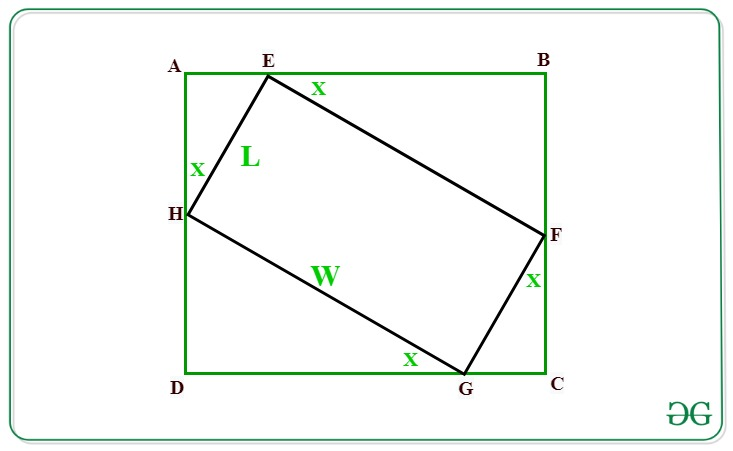# Maximum area of a Rectangle that can be circumscribed about a given Rectangle of size LxW

Given a rectangle of dimensions L and W. The task is to find the maximum area of a rectangle that can be circumscribed about a given rectangle with dimensions L and W.

Examples:

Input: L = 10, W = 10
Output: 200

Input: L = 18, W = 12
Output: 450

Approach: Let below is the given rectangle EFGH of dimensions L and W. We have to find the area of rectangle ABCD which is circumscribing rectangle EFGHIn the above figure:
Ifthenas GCF is right angled triangle.
Therefore,=>=>Similarly,Now, The area of rectangle ABCD is given by:

Area = (AE + EB)*(AH + HD) …..(1)

According to the projection rule:
AE = L*sin(X)
EB = W*cos(X)
AH = L*cos(X)
HD = W*sin(X)

Substituting the value of the above projections in equation (1) we have:Now to maximize the area, the value of sin(2X) must be maximum i.e., 1.
Therefore after substituting sin(2X) as 1 we have,Below is the implementation of the above approach:

## C++

 // C++ program for the above approach  #include  using namespace std;    // Function to find area of rectangle  // inscribed another rectangle of  // length L and width W  double AreaofRectangle(int L, int W) {           // Area of rectangle      double area = (W + L) * (W + L) / 2;           // Return the area      return area; }   // Driver Code  int main()  {            // Given dimensions      int L = 18;     int W = 12;           // Function call      cout << AreaofRectangle(L, W);     return 0;  }    // This code is contributed by Princi Singh

## Java

 // Java program for the above approach  import java.io.*; import java.util.*;    class GFG{       // Function to find area of rectangle  // inscribed another rectangle of  // length L and width W  static double AreaofRectangle(int L, int W) {           // Area of rectangle      double area = (W + L) * (W + L) / 2;           // Return the area      return area; }       // Driver Code  public static void main(String args[]) {            // Given dimensions      int L = 18;     int W = 12;           // Function call      System.out.println(AreaofRectangle(L, W)); }  }    // This code is contributed by offbeat

## Python3

 # Python3 program for the above approach   # Function to find area of rectangle  # inscribed another rectangle of  # length L and width W def AreaofRectangle(L, W):       # Area of rectangle   area =(W + L)*(W + L)/2   # Return the area   return area   # Driver Code if __name__ == "__main__":     # Given Dimensions   L = 18   W = 12     # Function Call   print(AreaofRectangle(L, W))

## C#

 // C# program for the above approach  using System;   class GFG{       // Function to find area of rectangle  // inscribed another rectangle of  // length L and width W  static double AreaofRectangle(int L, int W) {           // Area of rectangle      double area = (W + L) * (W + L) / 2;           // Return the area      return area; }       // Driver Code  public static void Main(String []args) {            // Given dimensions      int L = 18;     int W = 12;           // Function call      Console.Write(AreaofRectangle(L, W)); }  }    // This code is contributed by shivanisinghss2110

## Javascript

 

Output:

450.0

Time Complexity: O(1)
Auxiliary Space: O(1)

Feeling lost in the world of random DSA topics, wasting time without progress? It's time for a change! Join our DSA course, where we'll guide you on an exciting journey to master DSA efficiently and on schedule.
Ready to dive in? Explore our Free Demo Content and join our DSA course, trusted by over 100,000 geeks!